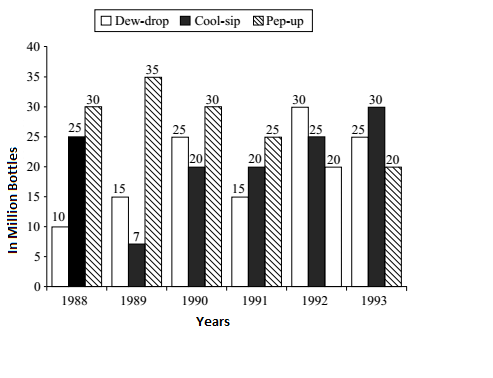## Introduction to Data Interpretation

#### Data Interpretation

Direction: Study the following graph carefully and answer the questions given below.1. What was the approximate % drop in sale of Pep-up in 1990 over its sale in 1989?

1.  Required % drop = Number of sale of Pep-up in 1989 - Number of sale of Pep-up in 1990 X 100 Number of sale of Pep-up in 1989

##### Correct Option: C

 Required % drop = Number of sale of Pep-up in 1989 - Number of sale of Pep-up in 1990 X 100 Number of sale of Pep-up in 1989

 Required % drop = 35 - 30 X 100 ≈ 14% 35

1. In the year 1990, what was the difference between the number of ‘Pep-up’ and ‘Cool-sip’ bottles sold?

1. Difference in Required number = Number of Pep-up drink sold in 1990 - Number of Cool-sip drink sold in 1990.

##### Correct Option: E

Difference in Required number = Number of Pep-up drink sold in 1990 - Number of Cool-sip drink sold in 1990.
Difference in Required number = 30 million − 20 million = 10 million.
Difference in Required number = 10 million = 10 x 1000000 ( Since 1 million = 1000000 )
Difference in Required number = 10 million = 10000000

1. In the case of Cool-sip drink, what was the approximate % increase in sale in 1992 over its sale in 1991?

1.  Required % = ( Number of Cool-sip drink in 1992 - Number of Cool-sip drink in 1991 ) X 100 Number of Cool-sip drink in 1991

##### Correct Option: C

 Required % = ( Number of Cool-sip drink in 1992 - Number of Cool-sip drink in 1991 ) X 100 Number of Cool-sip drink in 1991

 Required % = 25 - 20 X 100 = 25% 20

1. In the case of which soft drink was the average annual sale maximum in the given period?

1. Avg. annual sale of Dew-drop

 = 10 + 15 + 25 + 15 + 30 + 25 = 20 Million 6

Similarly calculate for the other drinks also.

##### Correct Option: A

Avg. annual sale of Dew-drop

 = 10 + 15 + 25 + 15 + 30 + 25 = 20 Million 6

Average anual sale of Cool-sip
 = 25 + 7 + 2C + 20 + 25 + 30 = 21.16 Million 6

Average annual sale of Pep-up
 = 30 + 35 + 30 + 25 + 30 = 26.66 Million 6

1. In which year was the sale of ‘Pep-up’ the maximum?

1. As per given graph we can compare the sale graph for Pep Up for all given years.

##### Correct Option: E

By comparing the Sale of Pep-up for all years and we found that the Sale of Pep-up was the maximum in the year 1989.